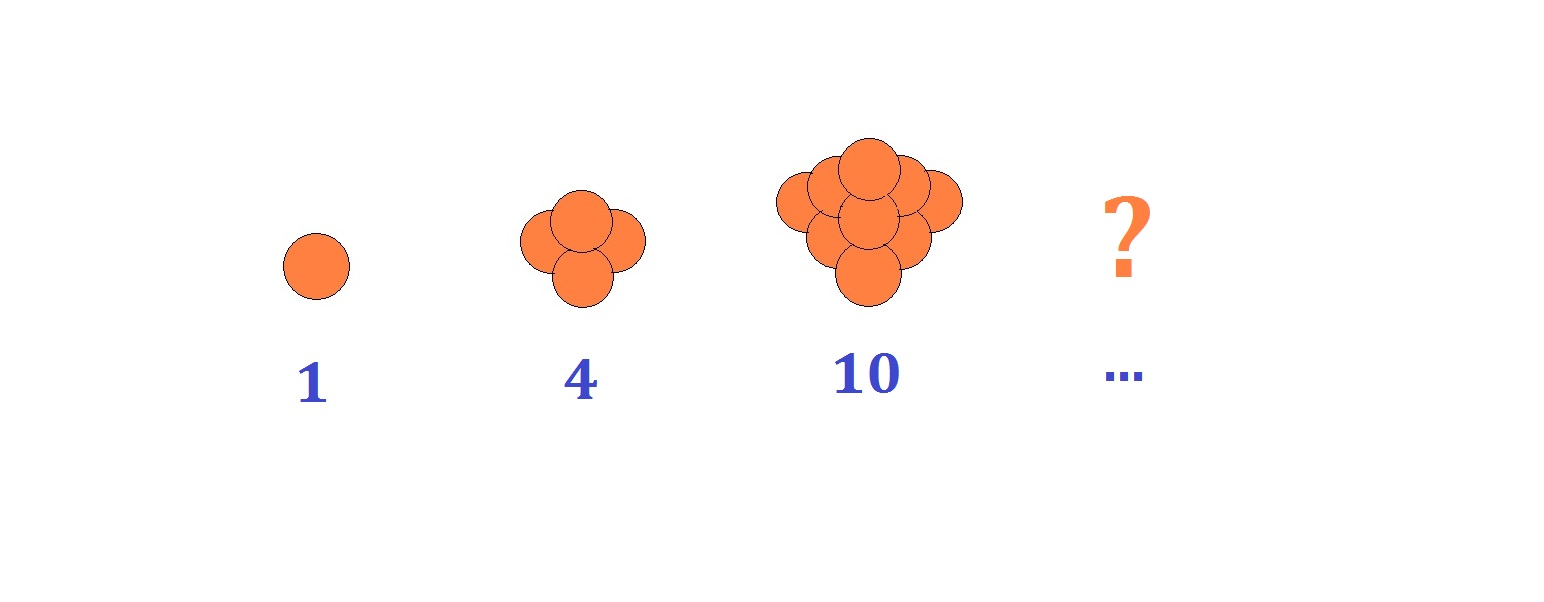Can you count all these oranges?

Probability Level 3The oranges are arranged so that there is 1 top orange; the second top layer has 2 more oranges than the top; the third has 3 more oranges than the second, and so on. Forming a tetrahedron of oranges, this "tetrahedral" number of oranges runs as a series as shown above.

What is the value of the $100^{\text{th}}$ term of this series?

×